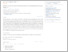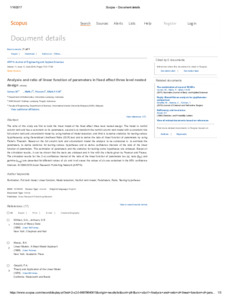# Analysis and ratio of linear function of parameters in fixed effect three level nested design

Usman, Mustofa and Malik, Ibnu and Warsono, Warsono and Elfaki, Faiz Ahmed Mohamed (2016) Analysis and ratio of linear function of parameters in fixed effect three level nested design. ARPN Journal of Engineering and Applied Sciences, 11 (11). pp. 7121-7129. ISSN 1819-6608PDF - Published Version
Restricted to Repository staff onlyPreview
PDF

## Abstract

The aims of this study are first to build the linear model of the fixed effect three level nested design. The model is not full column rank and has a constraint on its parameters; second is to transform the non full column rank model with a constraint into full column rank and unconstraint model by using method of model reduction; and third is to derive statistics for testing various hypotheses by using Generalized Likelihood Ratio (GLR) test and to derive the ratio of linear function of parameters by using Fieller’s Theorem . Based on the full column rank and unconstraint model the analysis to be conducted is : to estimate the parameters, to derive statistics for testing various hypotheses and to derive confidence intervals of the ratio of the linear function of parameters. The estimation of parameters and the statistics for testing some hypotheses are unbiased. Based on the simulation results, it can be shown that the tests are unbiased and in line with the criteria given by Pearson and Please. The simulation results for the (1-α) confidence interval of the ratio of the linear function of parameters tau (τi), beta (βj(i)) and gamma (γk(ij)) are presented for different values of ρ’s and in all cases the values of ρ’s are contained in the 95% confidence intervals.

Item Type: Article (Journal) 4925/51057 nonfull rank model, full rank model, model reduction, estimation, testing hypotheses, ratio, linear function, parameters Q Science > QA Mathematics Kulliyyah of Engineering > Department of Science Dr faiz elfaki 13 Jul 2016 06:19 16 Jan 2017 14:46 http://irep.iium.edu.my/id/eprint/51057View Item